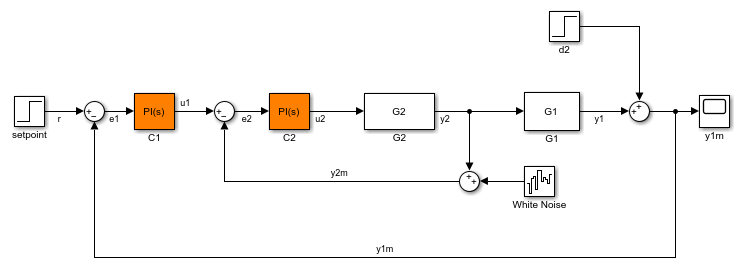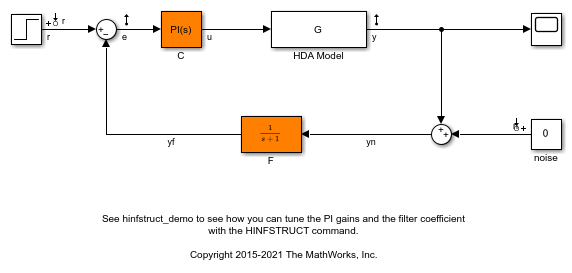# setTunedValue

Set current value of tuned variable in `slTuner` interface

## Syntax

``setTunedValue(st,var,value)``
``setTunedValue(st,varValues)``
``setTunedValue(st,model)``

## Description

`setTunedValue` lets you initialize or modify the current value of a tuned variable within an `slTuner` interface.

An `slTuner` interface parameterizes each tuned block as a Control Design Block, or a generalized parametric model of type `genmat` or `genss`. This parameterization specifies the tuned variables for commands such as `systune`.

example

````setTunedValue(st,var,value)` sets the current value of the tuned variable, `var`, in the `slTuner` interface, `st`.```

example

````setTunedValue(st,varValues)` sets the values of multiple tuned variables in `st` using the structure, `varValues`.```

example

````setTunedValue(st,model)` updates the values of the tuned variables in `st` to match their values in the generalized model `model`. To propagate tuned values from one model to another, use this syntax.```

## Examples

collapse all

Create an `slTuner` interface for the `scdcascade` model.

```open_system('scdcascade') st = slTuner('scdcascade',{'C1','C2'}); ```Set a custom parameterization for one of the tunable blocks.

```C1CustParam = realp('Kp',1) + tf(1,[1 0]) * realp('Ki',1); setBlockParam(st,'C1',C1CustParam); ```

These commands set the parameterization of the `C1` controller block to a generalized state-space (`genss`) model containing two tunable parameters, `Ki` and `Kp`.

Initialize the value of `Ki` to `10` without changing the value of Kp.

```setTunedValue(st,'Ki',10); ```

Create an `slTuner` interface for the `scdcascade` model.

```open_system('scdcascade') st = slTuner('scdcascade',{'C1','C2'}); ```Set a custom parameterization for one of the tunable blocks.

```C1CustParam = realp('Kp',1) + tf(1,[1 0]) * realp('Ki',1); setBlockParam(st,'C1',C1CustParam); ```

These commands set the parameterization of the `C1` controller block to a generalized state-space (`genss`) model containing two tunable parameters, `Ki` and `Kp`.

Create a structure of tunable element values, setting `Kp` to `5` and `Ki` to `10`.

```S = struct('Kp',5,'Ki',10); ```

Set the values of the tunable elements in `st`.

```setTunedValue(st,S); ```

Convert an `slTuner` interface for the Simulink® model `rct_diskdrive` to a `genss` model to tune the model blocks using `hinfstruct`. After tuning, update the `slTuner` interface with the tuned parameters and write the parameter values to the Simulink model for validation.

Use of `hinfstruct` requires a Robust Control Toolbox license.

Create an `slTuner` interface for `rct_diskdrive`. Add `C` and `F` as tuned blocks of the interface.

```open_system('rct_diskdrive'); st = slTuner('rct_diskdrive',{'C','F'}); ```The default parameterization of the transfer function block, `F`, is a transfer function with two free parameters. Because `F` is a low-pass filter, you must constrain its coefficients. To do so, specify a custom parameterization of `F` with filter coefficient `a`.

```a = realp('a',1); setBlockParam(st,'F',tf(a,[1 a])); ```

Convert `st` to a `genss` model.

```m = getIOTransfer(st,{'r','n'},{'y','e'}); ```

Typically, for tuning with `hinfstruct`, you append weighting functions to the `genss` model that depend on your design requirements. You then tune the augmented model. For more information, see Fixed-Structure H-infinity Synthesis with hinfstruct (Robust Control Toolbox).

For this example, instead of tuning the model, manually adjust the tuned variable values.

```m.Blocks.C.Kp.Value = 0.00085; m.Blocks.C.Ki.Value = 0.01; m.Blocks.a.Value = 5500; ```

After tuning, update the block parameterization values in `st`.

```setTunedValue(st,m); ```

This is equivalent to `setBlockValue(st,m.Blocks)`.

To validate the tuning result in Simulink, first update the Simulink model with the tuned values.

```writeBlockValue(st); ```

## Input Arguments

collapse all

Interface for tuning control systems modeled in Simulink, specified as an `slTuner` interface.

Tuned variable within `st`, specified as a character vector or string. A tuned variable is any Control Design Block, such `realp`, `tunableSS`, or `tunableGain`, involved in the parameterization of a tuned Simulink block, either directly or through a generalized parametric model. To get a list of all tuned variables within `st`, use `getTunedValue(st)`.

`var` can refer to the following:

• For a block parameterized by a Control Design Block, the name of the block. For example, if the parameterization of the block is

`C = tunableSS('C')`

then set `var` = `'C'`.

• For a block parameterized by a `genmat`/`genss` model, `M`, the name of any Control Design Block listed in `M.Blocks`. For example, if the parameterization of the block is

```a = realp('a',1); C = tf(a,[1 a]);```

then set `var` = `'a'`.

Value of tuned variable in `st`, specified as a numeric scalar, a numeric array or a state-space model that is compatible with the tuned variable. For example, if `var` is a scalar element such as a PID gain, `value` must be a scalar. If `var` is a 2–by–2 `tunableGain`, then `value` must be a 2–by–2 scalar array.

Values of multiple tuned variables in `st`, specified as a structure with fields specified as numeric scalars, numeric arrays, or state-space models. The field names are the names of tuned variables in `st`. Only blocks common to `st` and `varValues` are updated, while all other blocks in `st` remain unchanged.

To specify `varValues`, you can retrieve and modify the tuned variable structure from `st`.

```varValues = getTunedValue(st); varValues.Ki = 10; ```

Tuned model that has some parameters in common with `st`, specified as a Generalized LTI Model. Only variables common to `st` and `model` are updated, while all other variables in `st` remain unchanged.

collapse all

### Tuned Blocks

Tuned blocks, used by the `slTuner` interface, identify blocks in a Simulink model whose parameters are to be tuned to satisfy tuning goals. You can tune most Simulink blocks that represent linear elements such as gains, transfer functions, or state-space models. (For the complete list of blocks that support tuning, see How Tuned Simulink Blocks Are Parameterized). You can also tune more complex blocks such as SubSystem or S-Function blocks by specifying an equivalent tunable linear model.

Use tuning commands such as `systune` to tune the parameters of tuned blocks.

You must specify tuned blocks (for example, `C1` and `C2`) when you create an `slTuner` interface.

`st = slTuner('scdcascade',{'C1','C2'})`

You can modify the list of tuned blocks using `addBlock` and `removeBlock`.

To interact with the tuned blocks use:

### Tuned Variables

Within an `slTuner` interface, tuned variables are any Control Design Blocks involved in the parameterization of a tuned Simulink block, either directly or through a generalized parametric model. Tuned variables are the parameters manipulated by tuning commands such as `systune`.

For Simulink blocks parameterized by a generalized model or a tunable surface:

For Simulink blocks parameterized by a Control Design Block, the block itself is the tuned variable. To modify the block value, you can use either `setBlockValue` or `setTunedValue`. Similarly, you can retrieve the block value using either `getBlockValue` or `getTunedValue`.

### Topics

Introduced in R2015b